# Top 4 reasons why to improve Power Factor

Top 4 reasons why to improve Power Factor 1024 576 Gaurav J

In this tutorial, we’ll learn about the top 4 reasons why we should improve power factor. We’ll also understand why we should try to maintain the power factor for the system or machineries near to unity (1). So, let’s start.

In the Previous video, we have seen the very basic concept of power factor. I strongly recommend you to go through that tutorial first and then come back to this, as it will help you to understand it more clearly. Already done? Then you are good to go.

Let’s say, I am building a steel plant. For which, I have brought a 200KVA transformer, which is capable to transfer 170 kW power. Similarly, I also brought 1 HP induction motor & other required equipment. Based on my calculation, I found that my normal current would be approx. 280 amps RMS. Therefore, I chose conductors, who are capable of carrying the normal current up to 300 A. So, after all the calculations, I installed the conductors and other equipment, and production in my plant started.

After one month, I received my electricity bill, which was much higher than what I have expected! When I was going through the details, I found that power factor of my system is 0.7! And because of that, the utility has charged me penalty. Now, let’s find out what is the effect of this power factor on my system.

## Reason 1

First of all, let’s see what will happen with my transformer. I brought the 200 KVA transformer to deliver 170 kW of power. But, as the PF is 0.7, total power delivered by my transformer is only 140 kW.

P = 200 X 0.7 = 140kW

So, here is the first reason why we should improve the power factor,

#1 Better utilisation of electrical machineries.

Because of the low power factor, the efficiency of my transformer is poor, and hence, there is a possibility that it’ll consume more power. The same thing is also applicable to generators. If I improve the power factor to 0.9, then the transformer will deliver 180 kW of power.

P = 200 X 0.9 = 180kW

And if I continue with 0.7 power factor, then I have to buy a 250KVA transformer to fulfil my load. look at the highlighted (red colour) section below.

So, in conclusion, better power factor will reduce the size of machines which will ultimately save the cost. Now let’s see what will happen with my induction motor. With 0.7 power factor my 1 HP induction motor will take 1.42 HP.
HP = 1/0.7 = 1.42

Now, it is consuming 0.42 HP extra from the utility. Of course, the utility will charge extra for that, which means high electricity bill.

Motor will consume only 1HP from the line, if the power factor is unity or near to unity. Therefore, saving in electricity bill.

## Reason 2

Now, coming to the conductor I chose to carry my normal current i.e. 280 amps. To carry this current I have chosen 70 sq.mm XLPE conductors. But as my power factor is 0.7, current flowing through my system must have been increased. So, let’s calculate the new current.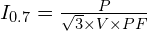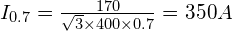As per the new calculations, the normal current is now 350 amps, which is more than what my conductors can carry normally. And hence it will cause heating of my conductors, as my conductors are over burdened. Also, life of it will reduce to approx. 50%. So, here is the reason number 2 for why we should improve power factor.

#2 Better utilisation of conductors.

If the pf would have been 0.9, then the normal current would have been 272 amps, but now it is 350 amps.

## Reason 3

As normal current is now increased, the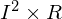losses are also increased. Which will further cause more power dissipation. Assuming resistance of system 0.94 ohms, with 0.7 power factor I sq. R losses are 115kW.

If I improve the power factor to 0.9, then the I sq. R losses will be reduced to 69.54 kW.

#### With 0.7 PF

Losses =Losses =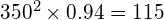KW

#### With 0.9 PF

Losses =Losses =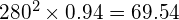KW

So, here is the reason number 3, why we should improve power factor.

#3 Reduction inlosses.

By improving the pf from 0.7 to 0.9, I can achieve savings in losses almost by 40%, and that’s a great saving.

## Reason 4

So, as we have seen with reduction in power factor, the system will start consuming more power form utility. And of course, for this extra power, utility will give penalties. In Maharashtra, one of the state in India, the utility gives penalty of about 10% of total bill amount, for pf less than or equal to 0.81.

So, if your electricity bill is 100,000 INR with power factor less than 0.81, you have to additionally pay 10,000 INR as penalty. So, the total bill is 110,000 INR. But, if you maintain the pf between 0.95 to 1, then the utility will give you incentive (reduction) of 7% on your total bill.

So, if the bill is 100,000 INR then you can save 7,000 INR per month, and 84,000 INR per year. The penalties & incentives may very state to state & country to country. So, here is the 4th reason, why we should improve power factor.

#4 To avoid penalties, and to get incentives from utilities.

## Summary

So, let’s take out the main points summarising why we should improve power factor.

1. For better utilisation of electrical machineries.
2. For better utilisation of conductors
3. To reduce the losses
4. To avoid penalties, and to get incentives from utilities.###### Gaurav J

Electrical Engineer. Content Creator. Currently working with a High & Extra High Voltage Switchgear Industry.

All stories by: Gaurav J